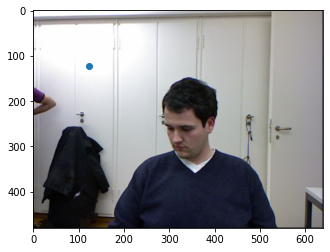# Fastbook Chp. 6 - Multiple PointBlocks

I’m working through fastbook chapter 6, using the Facial Keypoints Dataset on Kaggle.

I’ve manipulated the data a little, converting the tensors to images and saving them down so I can use transforms later. When I use the following DataBlock, everything works correctly and trains well.

data = DataBlock(
blocks=(ImageBlock, PointBlock),
)

However, when I try to add additional PointBlocks I get some errors. The DataBlock runs fine, so does the subsequent set up of Dataloaders. `dls.show_batch()` also runs, and shows the points in the correct positions.

`xb,yb = dls.one_batch()` gives the following error: `ValueError: too many values to unpack (expected 2)`

When I try to train I get the error `TypeError: 'L' object is not callable`

Here is the troublesome DataBlock set up…

``````data = DataBlock(
blocks=(ImageBlock, PointBlock, PointBlock, PointBlock),
n_inp=1
)
``````

I feel like i’m making a simple error in the way i’m structuring `get_y`, but after much googling and trial and error, i’ve been unable to make progress. Any help much appreciated.

2 Likes

One single PointBlock works on any number of points. For example see the `cats` dataset (from Kaggle). I made an example notebook here. We identify 9 points in each image (and only one `PointBlock` is used)

3 Likes

Thanks very much (as always!) @muellerzr, i’ll take a look at the notebook and try to work back from that.1 Like

No problem @PDiTOThe key is inside the `get_y` function, you should have it return a list of `x,y`. IIRC in v1 it wanted `y,x` unless you enabled something but this isn’t a thing in v2 (you can check the notebook)

But for instance this should be the output of your `get_y`:

``````tensor([[563., 411.],
[736., 404.],
[669., 545.],
[404., 340.],
[380., 148.],
[528., 261.],
[739., 254.],
[869., 123.],
[811., 325.]])
``````

Now the order will matter here, and if there are missing values I’d recommend putting them in a place you know already, such as maybe -1,-1 (this is done with object detection to define a point is missing)

1 Like

Thanks again @muellerzr. It worksand I now understand what get_y expects better!

For anyone interested, my solution that now trains looks like:-

``````def get_y(r):
return [
[r['nose_tip_x'],r['nose_tip_y']],
[r['left_eye_center_x'],r['left_eye_center_y']],
[r['right_eye_center_x'],r['right_eye_center_y']],
]

data = DataBlock(
blocks=(ImageBlock, PointBlock),
get_y=get_y
)``````
4 Likes

Just in time for my work. Thanks.

Hi everyone,

I am having difficulties in interpreting the predictions from the learner object, with the original problem described in Chapter 6, when I run predict on one of the images from the dataset, I get

``````db = DataBlock(blocks=(ImageBlock, PointBlock),
get_items=get_image_files,
get_y= get_ctr,
splitter=FuncSplitter(lambda fn: fn.parent.name == "01"),
item_tfms=Resize(224),
batch_tfms=aug_transforms() # this is different to that described in the book.
)
ds = db.datasets(path)
learn = cnn_learner(dls, resnet18, y_range=(-1,1))
# steps to train
# ....
# ...

# get one image from the dataset
img = ds

# make a prediction
learn.predict(img)
# output:
#   (TensorPoint([[123.3277, 121.6380]]),
#    tensor([0.1011, 0.0861]),
#    tensor([0.1011, 0.0861]))
``````

How can I reconstruct the point on the image from this output?
While using the first TensorPoint (123.3277, 121.6380), it is going way off the face, while `learn.show_results()` works basically fine!

``````plt.imshow(img)
plt.scatter(123.3277, 121.6380)
``````Thanks,
Sam

I believe the result is based on the resized image (224x224) so the prediction of (123, 121) seems reasonable. Therefore, to use the original image, you would need to scale the prediction.

This link may help with a solution: https://youtu.be/pQ7CJzGn6YE?t=3553

To rescale the prediction output to the original image, the point in encoded coordinates can be decoded with a `PointScaler`:

``````img, ref_point = ds
_,_,p = learn.predict(img) # p = tensor([0.1011, 0.0861])
sclr = PointScaler()
sclr(img) # Transforming the image stores the image size in self.sz
dp = sclr.decode(TensorPoint.create(p)) # need a TensorPoint
print(dp, ref_point)
``````